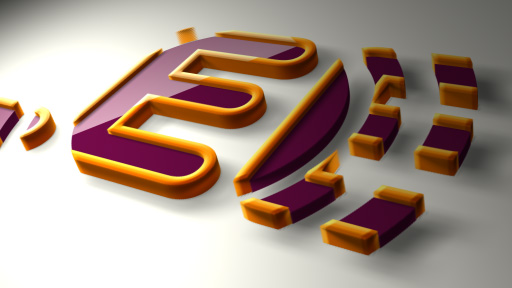# Layer distribute in z space in relation to layer number

[value, value, value-index]

# Bounce Script

Here is a very handy AE script for making you graphics bounce when they scale or move in.

amp = .1;

freq = 2.0;

decay = 2.0;

n = 0;

if (numKeys > 0){n = nearestKey(time).index;if (key(n).time > time){n–;}}

if (n == 0){ t = 0;}else{t = time – key(n).time;}

if (n > 0){v = velocityAtTime(key(n).time – thisComp.frameDuration/10);

value + v*amp*Math.sin(freq*t*2*Math.PI)/Math.exp(decay*t);

}else{value}

Change the values of the amp, freq and decay to change the speed and amount of bounce.

# Lens Flare Track

This lovely little script tracks the movement of an element in 3D space and maps it onto the position of you lens flare.

L = thisComp.layer(“Flare”);L.toComp([0,0,0]);

Just change the name of the layer you want to track to Flare.

# Match camera focal plane to another layer

target = thisComp.layer(“target”);
V1 = target.toWorld(target.anchorPoint) – toWorld([0,0,0]);
V2 = toWorldVec([0,0,1]);
dot(V1,V2);

# Fade opacity of a 3D layer based on distance from camera

try{ // Check whether there’s a camera.
C = thisComp.activeCamera.toWorld([0,0,0]);
}catch(err){ // No camera, so assume 50mm.
w = thisComp.width * thisComp.pixelAspect;
C = [0,0,-z];
}
P = toWorld(anchorPoint);
d = length(C,P);

# Loop

loopOut(type = “cycle”)

loopOut(type = “pingpong”)

loopOutDuration(type = “cycle”, duration = 1)

Change the duration to add a gap

# Clock Hands

thisComp.layer(“Deep Red Solid 2”).transform.zRotation/12

jjgifford

graymachine my top expressions

motionscript